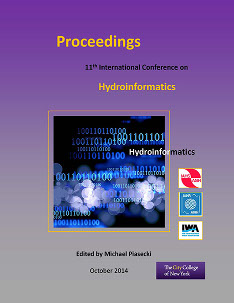International Conference on Hydroinformatics

Presentation

8-1-2014

Abstract

The effective management of groundwater systems relies on the adequate knowledge of its hydro-geological parameters. In large aquifer systems, it is often computationally expensive to estimate the spatially distributed aquifer parameters. Inverse modeling of these parameters are usually required in simulation of flow and contaminant transport in the problem domain for its meaningful system prediction. In the present study, a new approach for inverse modeling is adopted based on Meshless Local Petrov Galerkin (MLPG) flow simulation model which is coupled with Particle Swarm Optimization (PSO) model. MLPG is one of the meshless techniques, which are recently developed to solve many partial differential governing equations in various engineering fields. Here, without using a pre-defined mesh, the system of equations are established for the entire domain. In MLPG, only appropriate distribution of nodes is utilized in the modeling. The nodes are used for approximation of the governing equations by using support domain. This alleviates the huge efforts required in pre-processing for groundwater modeling, as in mesh based methods. The numerical model is developed in 2 dimensions using MATLAB. The standard PSO algorithm is used for optimization and both simulation optimization models are coupled. The model is applied to a hypothetical confined aquifer to compute transmissivity in different zones of the aquifer. The stability of the estimated parameter is investigated by considering different sets of head data, assuming error free head and different sets involving measurement errors. The solutions are compared with other inverse models using the Levenberg-Marquardt Algorithm (LMA) and Genetic Algorithm (GA). The PSO results are comparable with LMA and are better compared to GA estimates. From the results we can say that the model can be applied to obtain optimal estimates of the aquifer parameters in the regional groundwater systems.